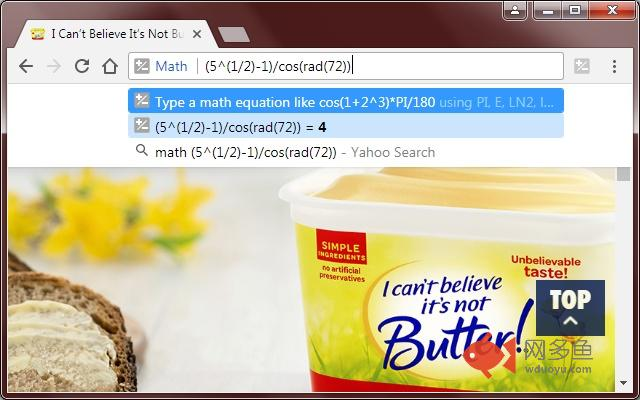# Math

2021年04月06日 搜索工具

### 插件截图### 插件概述

Solve math equations in the address bar by typing 'math' then something like: (sqrt(5)-1)/cos(rad(72))

### 插件详情

First type "math", then an empty space, then the equation. The answer will appear in the drop down menu.

You can type trigonometry too like:

math 10 + cos(rad(45)) + log(10) + PI*180 / (2^4 + atan(1))

If you press the "enter" key the result will be saved and is accessible by clicking on the toolbar icon. Only the last 30 calculations will be saved and they will be cleared when the browser is closed.

Note that (^) is a "the power of" operator, and not a bitwise-or operator.
And that (~) is the "negative" operator, so negative 2 is "~2" and not "-2".

Supported constants are:
Euler's constant (E), Natural log of 2 (LN2), Natural log of 10 (LN10), Base 2 log of E (LOG2E), Base 10 log of E (LOG10E), Pi (PI), Square root of 0.5 (SQRT1_2), Square root of 2 (SQRT2)

Supported (one argument) functions are:
abs(x), acos(x), acosh(x), asin(x), asinh(x), atan(x), atanh(x), cube root (cbrt(x)), cos(x), cosh(x), radians to degrees (deg(x)), E to the power of x (exp(x)), log(x), log10(x), log2(x), degrees to radians (rad(x)), sin(x), sinh(x), square root (sqrt(x)), tan(x), tanh(x).

### 插件下载

 下载链接一     下载链接二 Chrome插件离线安装教程